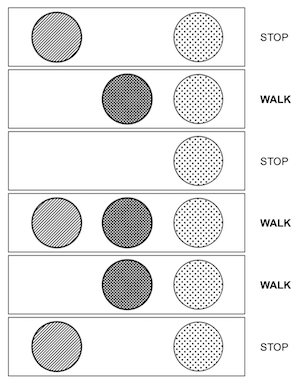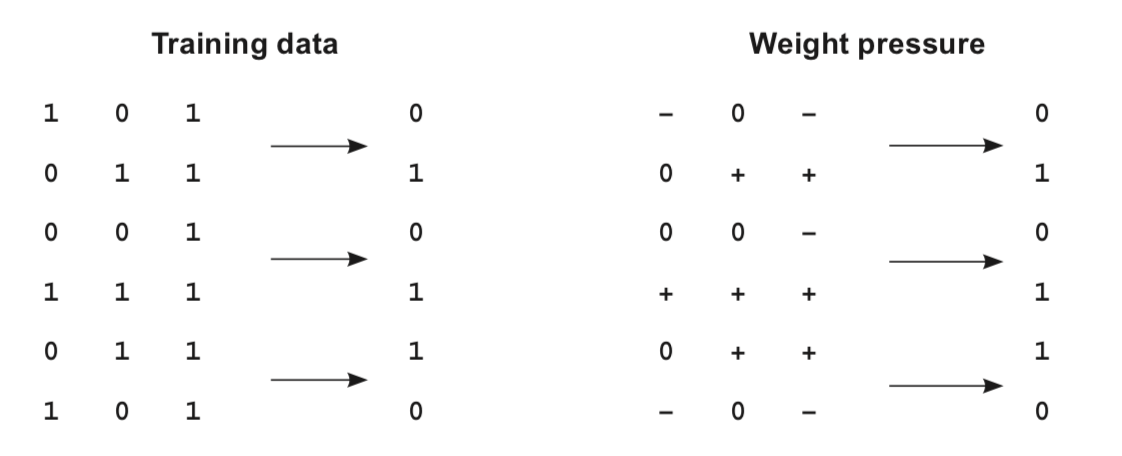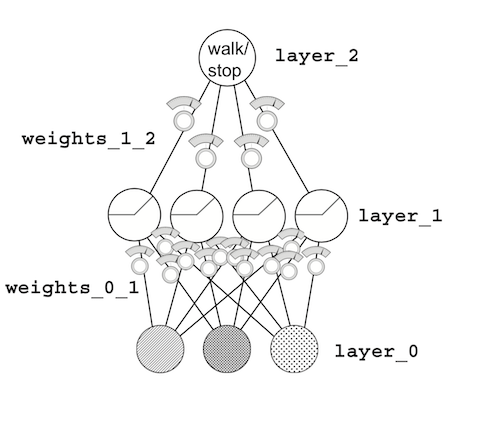# Building a deep neural network

These are my notes from the book Grokking Deep Learning by Andrew Trask. Feel free to check my first post on this book to get my overall thoughts and recommendations on how to approach this series. The rest of my notes for this book can be found here

### Streetlights example.

The pattern of the streetlights correlates to some output indicating whether to walk or stop.Turn the pattern of lights into ones and zeros, 1=on, 0=off. Also the output, 1=walk 0=stop.

This can be written in a matrix format.

# An input matrix of streetlight patterns
streetlights = np.array([[1, 0, 1],
[0, 1, 1],
[0, 0, 1],
[1, 1, 1],
[0, 1, 1],
[1, 0, 1]])

# Output data for whether pattern means walk vs stop.
walk_vs_stop = np.array([,
,
,
,
,
])


The data matrix doesn’t have to always be ones and zeros. The lights can be dimmers, with varying degrees of intensity.

# Varying degrees of intensity
streetlights_dimmer = np.array([[.9, .0, 1],
[.2, .8, 1],
[.1, .0, 1],
[.8, .9, 1],
[.1, .7, 1],
[.9, .1, 0]])

# Can also be scalar multiples, containing same pattern
streetlights_multiple = streetlights_dimmer * 10
streetlights_multiple

array([[ 9.,  0., 10.],
[ 2.,  8., 10.],
[ 1.,  0., 10.],
[ 8.,  9., 10.],
[ 1.,  7., 10.],
[ 9.,  1.,  0.]])

# Another example
streetlights_multiple = streetlights_dimmer * 20
streetlights_multiple

array([[18.,  0., 20.],
[ 4., 16., 20.],
[ 2.,  0., 20.],
[16., 18., 20.],
[ 2., 14., 20.],
[18.,  2.,  0.]])


The underlying pattern is a property of the matrix. It’s the pattern expressed in the streetlights. This input pattern is what we want the network to learn to transform into the output pattern.

### Building at neural network

Build a neural network using the streetlight matrix input and output

weights = np.array([0.5, 0.48, -0.7])
alpha = 0.1 # learning rate

streetlights = np.array([[1, 0, 1],
[0, 1, 1],
[0, 0, 1],
[1, 1, 1],
[0, 1, 1],
[1, 0, 1]])

walk_vs_stop = np.array([0, 1, 0, 1, 1, 0])

my_input = streetlights #[1, 0, 1]
goal_prediction = walk_vs_stop # Shoudl be 0 ie 'stop'

for iteration in range(20):
prediction = my_input.dot(weights)
error = (goal_prediction - prediction) ** 2
delta = prediction - goal_prediction
weights = weights - (alpha * (my_input * delta))

print('Error: ' + str(error) + ' Prediction:' + str(prediction))

Error: 0.03999999999999998 Prediction:-0.19999999999999996
Error: 0.025599999999999973 Prediction:-0.15999999999999992
Error: 0.01638399999999997 Prediction:-0.1279999999999999
Error: 0.010485759999999964 Prediction:-0.10239999999999982
Error: 0.006710886399999962 Prediction:-0.08191999999999977
Error: 0.004294967295999976 Prediction:-0.06553599999999982
Error: 0.002748779069439994 Prediction:-0.05242879999999994
Error: 0.0017592186044416036 Prediction:-0.04194304000000004
Error: 0.0011258999068426293 Prediction:-0.03355443200000008
Error: 0.0007205759403792803 Prediction:-0.02684354560000002
Error: 0.0004611686018427356 Prediction:-0.021474836479999926
Error: 0.0002951479051793508 Prediction:-0.01717986918399994
Error: 0.00018889465931478573 Prediction:-0.013743895347199997
Error: 0.00012089258196146188 Prediction:-0.010995116277759953
Error: 7.737125245533561e-05 Prediction:-0.008796093022207963
Error: 4.951760157141604e-05 Prediction:-0.007036874417766459
Error: 3.169126500570676e-05 Prediction:-0.0056294995342132115
Error: 2.028240960365233e-05 Prediction:-0.004503599627370569
Error: 1.298074214633813e-05 Prediction:-0.003602879701896544
Error: 8.307674973656916e-06 Prediction:-0.002882303761517324


### Learning the whole dataset

Previous was just a single streetlight example. Now lets train against all the lights at once.

weights = np.array([0.5, 0.48, -0.7])
alpha = 0.1 # learning rate

streetlights = np.array([[1, 0, 1],
[0, 1, 1],
[0, 0, 1],
[1, 1, 1],
[0, 1, 1],
[1, 0, 1]])

walk_vs_stop = np.array([0, 1, 0, 1, 1, 0])

my_input = streetlights #[1, 0, 1]
goal_prediction = walk_vs_stop # Shoudl be 0 ie 'stop'

for iteration in range(40):
error_for_all_lights = 0
for row_index in range(len(walk_vs_stop)):
my_input = streetlights[row_index]
goal_prediction = walk_vs_stop[row_index]

prediction = my_input.dot(weights)
error = (goal_prediction - prediction) ** 2
error_for_all_lights += error

delta = prediction - goal_prediction
weights = weights - (alpha * (my_input * delta))

print('Error: ' + str(error) + ' Prediction:' + str(prediction))
print('Error For All: ' + str(error_for_all_lights) + '\n')

Error: 0.03999999999999998 Prediction:-0.19999999999999996
Error: 1.44 Prediction:-0.19999999999999996
Error: 0.31359999999999993 Prediction:-0.5599999999999999
Error: 0.14745599999999992 Prediction:0.6160000000000001
Error: 0.6842598400000001 Prediction:0.17279999999999995
Error: 0.030807270400000003 Prediction:0.17552
Error For All: 2.6561231104

Error: 0.019716653055999997 Prediction:0.14041599999999999
Error: 0.48073921463296004 Prediction:0.3066464
Error: 0.11912040471029758 Prediction:-0.34513824
Error: 4.4054335374336594e-05 Prediction:1.006637344
Error: 0.2719586253784771 Prediction:0.4785034751999999
Error: 0.07129122555848956 Prediction:0.26700416768
Error For All: 0.9628701776715985

...

Error: 5.705876310825877e-06 Prediction:-0.002388697618122871
Error: 5.2801809843946525e-06 Prediction:0.9977021355600483
Error: 0.0003218187196733748 Prediction:-0.01793930655497516
Error: 0.00026288646758276113 Prediction:1.0162137740080082
Error: 1.0805129612468648e-05 Prediction:0.9967128843019345
Error: 7.847193319322342e-06 Prediction:-0.0028012842268006904
Error For All: 0.0006143435674831474

Error: 5.022203724366299e-06 Prediction:-0.0022410273814405524
Error: 4.517586152808343e-06 Prediction:0.9978745386023716
Error: 0.00027960068413413554 Prediction:-0.016721264429884947
Error: 0.00022839509133955668 Prediction:1.0151127459893812
Error: 9.307331052303591e-06 Prediction:0.9969492081270097
Error: 6.893876881709479e-06 Prediction:-0.0026256193329783125
Error For All: 0.00053373677328488


### Full, batch, and stochastic gradient descent

Stochastic - Updates weights one example at a time. Thats what was used in the example above. Performs a prediction and weight update for each training example separately. Moves through streetlights one at a time. Iterates through many times until it finds the best weight that works well for all examples.

Full - Rather than updating weights for each example, calculates the average weight_delta over entire dataset. Only changes weights each time it computes full average.

Batch - Splits the difference between full and stochastic. Set batch size (typically between 8 and 256) examples. Weights are updated after each batch.

### Up and down pressure

Error attribution - given a shared error, the network needs to figure out which weights contributed to the error, and which weights did not contribute. The ones that contribute get adjusted, the ones that do not get left alone.In first example above [1, 0, 1], the middle weight is irrelevent because the input is 0. No matter what the weight is, when it’s multiplied by 0, it will be 0. Thus any error from the training example must be attributed to either the first or third weights.

If the network should predict a 0, and the two inputs are 1’s, then the ‘pressure’ shoudl be pushing the weights for these two inputs down. Driving the weight values towards 0.

Since the prediction is a weighted sum (dot product of inputs & weights), the algorithm rewards inputs that correlated with output (upward pressure), and penalizes inputs with discorrelation (with downward pressure).

### Edge case: Overfitting

Again looking at the example above - what if the far left weight was 0.5, and the far right weight was -0.5? The prediction would be 0. This is a case where the network is predicting perfectly, but it’s not the correct prediction.

Overfitting is when the error is shared among the weights, and they accidentally create a perfect correlation, without giving the heaviest weight to the best inputs. When error==0, the neural network stops learning.

That’s why it is important to train on many examples. Too few, and the network will just memorize rather than generalize. In the case of the streetlights, it would just memorize that specific pattern, and not find the correlation that allows the network to generalize to any possible streetlight config.

Greatest challenge is convincing network to generalize instead of just memorize

From Fast.ai: It’s not easy to get your model to overfit. This can happen if you train for too long ie… too many epochs. A sign of this is when your error goes down, but then starts going up again.

A correctly trained model should have a lower train loss than validation loss

### Edge case: Conflicting pressure

Regularization aims to say that only weights with really strong correlation stay on. Everything else should be silenced because it’s contributing noise.

Regularization forces weights with conflicting pressure to move toward 0. If the weight does not have strong correlation, then try to turn it down so it doesn’t mess with the others.

If no correlation between any input column and the output column, then every weight has an equal amount of upward and downward pressure. This dataset is a challenge for neural networks.

### Learning indirect correlation

Neural network searches for correlation between input and output layers.

If input dataset does not correlate with output dataset, then add an intermediate dataset that does have correlation. Aka. hidden layer

### Your first deep neural network# Making a prediction
import numpy as np
np.random.seed(1)

# This function sets all negative numbers to 0
def relu(x):
return (x > 0) * x # if x > 0 return x, else return 0

alpha = 0.2
hidden_size = 4
streetlights = np.array([[1, 0, 1],
[0, 1, 1],
[0, 0, 1],
[1, 1, 1]])

walk_vs_stop = np.array([[1, 1, 0, 0]]).T

# Two sets of weights connected to 3 layers
# Randomly initialized
weights_0_1 = 2 * np.random.random((3, hidden_size)) - 1
weights_1_2 = 2 * np.random.random((hidden_size, 1)) - 1

layer_0 = streetlights
layer_1 = relu(np.dot(layer_0, weights_0_1))
layer_2 = np.dot(layer_1, weights_1_2)


Let’s break it down step by step

# Initalizes the weights for layer_0 to layer_1
# Should be 12 weights, since 3 nodes for input layer x 4 nodes for hidden
weights_0_1 = 2 * np.random.random((3, hidden_size)) - 1
weights_0_1

array([[-0.1653904 ,  0.11737966, -0.71922612, -0.60379702],
[ 0.60148914,  0.93652315, -0.37315164,  0.38464523],
[ 0.7527783 ,  0.78921333, -0.82991158, -0.92189043]])

# Initalizes weights for layer_1 to layer_2
# Should be 4 weights. 4 for hidden x 1 for output
weights_1_2 = 2 * np.random.random((hidden_size, 1)) - 1
weights_1_2

array([[-0.66033916],
[ 0.75628501],
[-0.80330633],
[-0.15778475]])

# First set of streetlight inputs
layer_0 = streetlights
layer_0

array([1, 0, 1])

# Takes dot product of layer_0 and weights_0_1
# Runs relu activation function to set negative values to 0
layer_1 = relu(np.dot(layer_0, weights_0_1))
layer_1

array([ 0.58738791,  0.90659298, -0.        , -0.        ])

# Prediction to output layer
# Dot product of layer_1 output and weights_1_2
layer_2 = np.dot(layer_1, weights_1_2)
layer_2

array([0.29776744])


### Backpropogation in code

import numpy as np

np.random.seed(1)

def relu(x):
return (x > 0) * x # returns x if x > 0
# return 0 otherwise

def relu2deriv(output):
return output>0 # returns 1 for input > 0
# return 0 otherwise

streetlights = np.array( [[ 1, 0, 1 ],
[ 0, 1, 1 ],
[ 0, 0, 1 ],
[ 1, 1, 1 ] ] )

walk_vs_stop = np.array([[ 1, 1, 0, 0]]).T

alpha = 0.2
hidden_size = 4

weights_0_1 = 2*np.random.random((3,hidden_size)) - 1
weights_1_2 = 2*np.random.random((hidden_size,1)) - 1

for iteration in range(60):
layer_2_error = 0
for i in range(len(streetlights)):
layer_0 = streetlights[i:i+1]
layer_1 = relu(np.dot(layer_0,weights_0_1))
layer_2 = np.dot(layer_1,weights_1_2)

layer_2_error += np.sum((layer_2 - walk_vs_stop[i:i+1]) ** 2)

layer_2_delta = (layer_2 - walk_vs_stop[i:i+1])
layer_1_delta=layer_2_delta.dot(weights_1_2.T)*relu2deriv(layer_1)

weights_1_2 -= alpha * layer_1.T.dot(layer_2_delta)
weights_0_1 -= alpha * layer_0.T.dot(layer_1_delta)

if(iteration % 10 == 9):
print("Error:" + str(layer_2_error))

Error:0.6342311598444467
Error:0.35838407676317513
Error:0.0830183113303298
Error:0.006467054957103705
Error:0.0003292669000750734
Error:1.5055622665134859e-05


### One iteration of backprop

Let’s break down each step.

Initialize network weights and data

import numpy as np

np.random.seed(1)
def relu(x):
return (x > 0) * x

def relu2deriv(output):
return output > 0

lights = np.array ([[1, 0, 1],
[0, 1, 1],
[0, 0, 1],
[1, 1, 1]])

walk_stop = np.array([[1, 1, 0, 1]]).T

alpha = 0.2
hidden_size = 3 # hidden layer with 3 nodes
weights_0_1 = 2 * np.random.random((3, hidden_size)) - 1
weights_1_2 = 2 * np.random.random((hidden_size, 1)) - 1


View initialized weights

walk_stop[0:1]

array([])

weights_0_1

array([[-0.16595599,  0.44064899, -0.99977125],
[-0.39533485, -0.70648822, -0.81532281],
[-0.62747958, -0.30887855, -0.20646505]])

weights_1_2

array([[ 0.07763347],
[-0.16161097],
[ 0.370439  ]])

layer_2_error = 0


Predict + Compare: Making prediction and calculate the output error and delta

layer_0 = lights[0:1]
layer_0

array([[1, 0, 1]])

layer_1 = relu(np.dot(layer_0, weights_0_1))
layer_1

array([[-0.        ,  0.13177044, -0.        ]])

# Prediction
layer_2 = np.dot(layer_1, weights_1_2)
layer_2

array([[-0.02129555]])

# Error (Same as Delta**2)
layer_2_error += np.sum((layer_2 - walk_vs_stop[0:1]) ** 2)
layer_2_error

1.0430445982842722

# Delta
layer_2_delta = (layer_2 - walk_vs_stop[0:1])
layer_2_delta

array([[-1.02129555]])


Learn: Backprop from layer_2 to layer_1

layer_1_delta = layer_2_delta.dot(weights_1_2.T)
layer_1_delta

array([[-0.07928672,  0.16505257, -0.3783277 ]])

layer_1_delta *= relu2deriv(layer_1)
layer_1_delta

array([[-0.        ,  0.16505257, -0.        ]])


Learn: Generate weight_deltas and update weights

weight_delta_1_2 = layer_1.T.dot(layer_2_delta)
weight_delta_1_2

array([[ 0.        ],
[-0.13457656],
[ 0.        ]])

weight_delta_0_1 = layer_0.T.dot(layer_1_delta)
weight_delta_0_1

array([[0.        , 0.16505257, 0.        ],
[0.        , 0.        , 0.        ],
[0.        , 0.16505257, 0.        ]])

weights_1_2 -= alpha * weight_delta_1_2
weights_1_2

array([[ 0.07763347],
[-0.13469566],
[ 0.370439  ]])

weights_0_1 -= alpha * weight_delta_0_1
weights_0_1

array([[-0.16595599,  0.40763847, -0.99977125],
[-0.39533485, -0.70648822, -0.81532281],
[-0.62747958, -0.34188906, -0.20646505]])


### Why do deep networks matter?

No individual pixel correlates with the final prediction… ie, whether or not the picture is of a cat. Each layer attempts to identify different configurations of pixels that may or may not correlate. The presence of many correlations, will give the final information the network needs to predict the correct output. Using the cat example, one layer could identify the edges of ears, the other could be the circles of eyes. Keep stacking these together and the final output will tell you whether the image overall correlates with that of a cat.